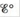# Direct methanol fuel cells (DMFCs) have shown some promise as a viable option for providing “green” energy to small electrical devices. Calculate for the reaction that takes place in DMFCs: CH 3 OH ( l ) + 3 / 2 O 2 ( g ) → CO 2 ( g ) + 2 H 2 O ( l ) Use values of Δ G f ∘ from Appendix 4.### Chemistry: An Atoms First Approach

2nd Edition
Steven S. Zumdahl + 1 other
Publisher: Cengage Learning
ISBN: 9781305079243

#### Solutions

Chapter
Section### Chemistry: An Atoms First Approach

2nd Edition
Steven S. Zumdahl + 1 other
Publisher: Cengage Learning
ISBN: 9781305079243
Chapter 17, Problem 56E
Textbook Problem
110 views

## Direct methanol fuel cells (DMFCs) have shown some promise as a viable option for providing “green” energy to small electrical devices. Calculatefor the reaction that takes place in DMFCs: CH 3 OH ( l )   + 3 / 2 O 2 ( g ) →  CO 2 ( g ) +   2 H 2 O ( l ) Use values of Δ G f ∘ from Appendix 4.

Interpretation Introduction

Interpretation:

The standard cell potential (Eο) for the given reaction that takes place in direct methanol fuel cell (DMFCs) is to be calculated.

Concept introduction:

The standard cell potential is defined as the electromotive force (emf) of a cell when a metal ion electrode is coupled with a hydrogen electrode under standard conditions and hence, is known as standard electrode potential.

To determine: The standard cell potential (Eο) for the given reaction takes place in direct methanol fuel cell (DMFCs).

### Explanation of Solution

The standard free energy of formation is shown below.

The given equation is,

CH3OH(l)+32O2(g)CO2(g)+2H2O(l) .

Refer Appendix 4.

The standard free energy of formation for the above reaction is,

SubstanceΔGfο(KJ/mol)CO2(g)394H2O(l)237CH3OH(l)166O2(g)0

The standard free energy of reaction is calculated by using the expression below by substituting the standard values of formation,

ΔGο=npΔGf(products)οnrΔGf(reactants)ο=ΔGf(CO2(g))ο+2ΔGf(H2O(l))οΔGf(CH3OH(l))ο(32)ΔGf(O2(g))ο

Substitute the required values in the above expression.

ΔGο=npΔGf(products)οnrΔGf(reactants)ο=ΔGf(CO2(g))ο+2ΔGf(H2O(l))οΔGf(CH3OH(l))ο(32)ΔGf(O2(

### Still sussing out bartleby?

Check out a sample textbook solution.

See a sample solution

#### The Solution to Your Study Problems

Bartleby provides explanations to thousands of textbook problems written by our experts, many with advanced degrees!

Get Started

Find more solutions based on key concepts
Why do some of these pressure belts occur in pairs?

Fundamentals of Physical Geography

Calorie deficit is no longer the primary strategy for weight management.

Nutrition: Concepts and Controversies - Standalone book (MindTap Course List)

What is the difference between hydrocarbons and other organic molecules?

Biology: The Dynamic Science (MindTap Course List)

Light can travel from air into water. Some possible paths for the light ray in the water are shown in Figure OQ...

Physics for Scientists and Engineers, Technology Update (No access codes included)

What tidal patterns are observed on the worlds coasts?

Oceanography: An Invitation To Marine Science, Loose-leaf Versin

How can some supernova remnants emit X-rays?

Foundations of Astronomy (MindTap Course List)